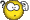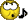# ALU WorksheetClassicListThreaded8 messagesOpen this post in threaded view
|

## ALU Worksheet

 I created a worksheet to help guide my students to realize that combining four simple Boolean/binary operations (zeroing, bitwise negation, summing, anding) in a two's complement number system can yield eighteen functions (per figure 2.6). This is to help assert the path of using the simple operations as mentioned in the implementation hint on page 39, as opposed to just diving in trying to implement the higher-level functions as they know them. For example, a student may try to use Inc16 to implement x+1 rather than using the combined simple operations in figure 2.6. Although this may be possible, I want to be sure the student embraces the underlying design of the ALU and has a nice "Aha!" moment. I'll let you know how well this works in practice in February 2010. alu_worksheet.pdf
Open this post in threaded view
|

## Re: ALU Worksheet

 Can you explain what I'm supossed to do in this worksheet? Im quite confused. Thank you.
Open this post in threaded view
|

## Re: ALU Worksheet

 I had the same problem at first. (That's why I ended up here.) After some time I finagled it out though: Each "exercise" in the worksheet corresponds to one line in the ALU-truth-table. So the first example, where the solution is given, corresponds to the line where f(x,y) is supposed to yield 0. The next where f(x,y) yields 1 ... and so on. (And rereading the text in the alu-worksheet it says so right from the beginning) BTW: Like probably many others in the forum I'm going through the book all on my own - with no background knowledge in EE or such. I therefore absolutely depend on the forum. Without you guys I probably would have been lost already in ch. 1. So - thanks a lot for all the summaries, helpful hints, tables and other tools you come up with!!!
Open this post in threaded view
|

## Re: ALU Worksheet

 In reply to this post by ybakos This worksheet was indeed great for seeing the patterns in the input bits to perform the various operations. However, the subtraction math just isn't clicking for me. For example, to perform x-y I immediately thought you would change it to x+(-y). In other words, you would make y negative and then add it to x. Instead, we modify x (negate it bitwise) add it to y, and then negate the answer. I don't see how this set of steps maps to the 2's complement math described in the book.
Open this post in threaded view
|

## Re: ALU Worksheet

Note: in what follows '~', '&' and '|' are binary operators NOT, AND, and OR. '-', '*' and '+' are arithmetic operators.

First, here's an identity for two's complement numbers that's very useful for proving ALU computations:
-x = (~x)+1
and a corollary:
~x = (-x)-1 = -(x+1)

Here's how x-y works. The ALU computation is zx=0, nx=1, zy=0, ny=0, f=1, no=1, so the ALU computes
out = ~(~x+y)

Here's the proof that out = x-y:

 out = ~(~x+y) = ~(-(x+1)+y) = ~(-x-1+y) = -(-x-1+y)-1 = x+1-y-1 out = x-y

--Mark

Open this post in threaded view
|

## Re: ALU Worksheet

 Mark, thanks a million for your quick and informative response.  The proof you provided for x-y could not have been clearer.  I never would have arrived at that myself, but it helps me a great deal to see it in action. If the authors of the book are reading this, please include the following info that Mark gave in the next edition. It would make the steps of the operations MUCH clearer. First, here's an identity for two's complement numbers that's very useful for proving ALU computations:     -x = (~x)+1 and a corollary:     ~x = (-x)-1 = -(x+1)